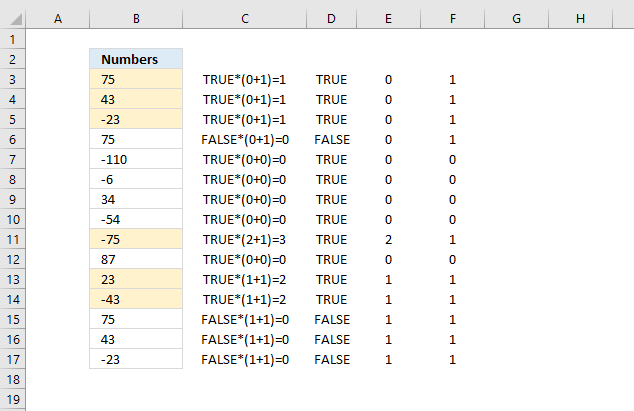Author: Oscar Cronquist Article last updated on October 31, 2018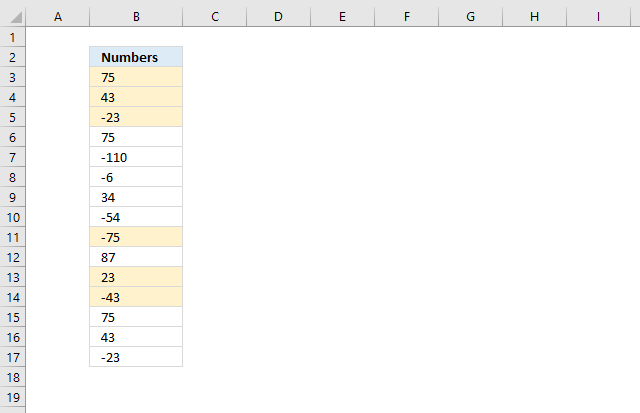I would like to ask you how to identify PAIR of same numbers, but with a different sign.
Ex. 1 with -1, 5000 with -5000, 75 with -75, etc.
Once I find those pairs, WITHOUT REPEATING
Ex. 75,-75,75, just the first TWO should get marked, and leave the last 75 alone.

Conditional formatting formula applied to cell range B3:B17:

=(COUNTIF(\$B\$3:B3, B3)=1)*(COUNTIF(\$B\$3:B3, B3*-1)+COUNT(MATCH(B3*-1, \$B\$3:\$B\$17, 0)))

### Explaining CF formula in cell B3

COUNTIF(\$B\$3:B3, B3)=1

The first COUNTIF function counts how many values there are in the first argument that match the second argument.

It returns TRUE if it is equal to 1, meaning this is the first instance of the value. In other words, this makes sure that duplicates are not highlighted.

The first argument is also a growing cell reference, the picture below shows what it returns in column D: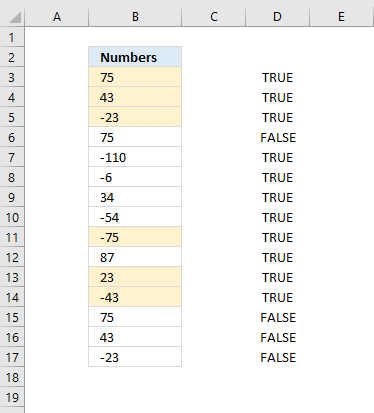COUNTIF(\$B\$3:B3, B3*-1)

The second COUNTIF function counts how many values there are in the first argument matching B3 but with a different sign, starting from the top.

This verifies that there is a number with a different sign above the current value.

The first argument has a growing cell reference meaning it expands as you copy the formula to cells below. In this case, I am using it as a Conditional Formatting formula, however, it behaves the same.

The picture below shows what it returns in column C: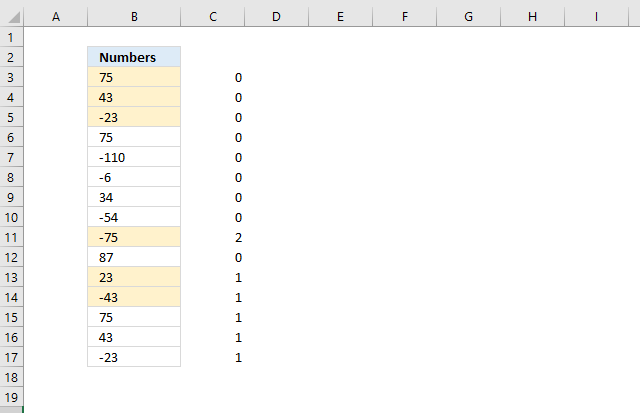MATCH(B3*-1,\$B\$3:\$B\$17,0)

The MATCH function looks for the number but with a different sign, this verifies it really exists a pair in the first place.

The MATCH function returns a #N/A error if a number is not found

COUNT(MATCH(B3*-1,\$B\$3:\$B\$17,0))

The COUNT function then converts error to a 0 (zero). The picture below shows what it returns in column E: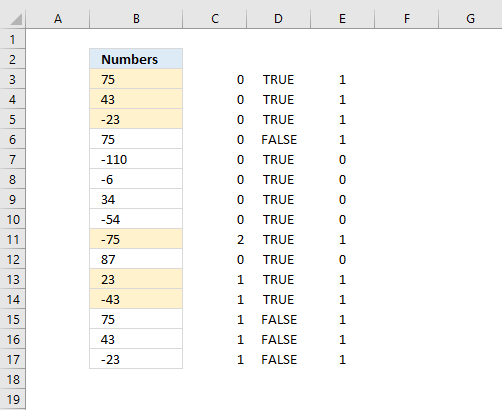Then the formula adds the numbers in the two last arrays, like this COUNTIF(\$B\$3:B3, B3*-1)+COUNT(MATCH(B3*-1, \$B\$3:\$B\$17, 0)

and lastly multiplies with COUNTIF(\$B\$3:B3, B3)=1.